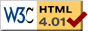### Journée-séminaire de combinatoire

#### (équipe CALIN du LIPN, université Paris-Nord, Villetaneuse)

Le 28 mars 2017 à 14h00 en B107, Sergey Dovgal nous parlera de : Phase Transition Threshold for Random Graphs and 2-SAT using Degree Constraints

Résumé : We show that by restricting the degrees of the vertices of a graph to an arbitrary set Δ, the threshold α(Δ) of the phase transition for a random graph with n vertices and m = α(Δ).n edges can be either accelerated (e.g., α(Δ) approx 0.38 for Δ = {0,1,4,5}) or postponed (e.g., α(Δ) approx 0.95 for Δ={1,2,50}) compared to a classical Erdős–Rényi random graph where α(N)=1/2. We investigate different graph statistics inside the critical window of transition (planarity, diameter, longest path...). We apply our results to a 2-SAT model with restricted literal degrees: the number of clauses that each literal is incident to belongs to the set Δ. We prove a lower bound for the probability that a formula with n variables and m=2.α(Δ) n clauses is satisfiable. This probability is close to 1 for the subcritical regime m=2.α.n.(1-μ.n-1/3), μ to ∞ and improves/generalizes the lower bound of Bollobás, Borgs, Chayes, Kim, and Wilson. This shows how the phase transition threshold for 2-SAT moves if we change the degrees of the literals. Joint work with Vlady Ravelomanana.

[arXiv]

 Dernière modification : Monday 24 January 2022Contact pour cette page : Cyril.Banderier at lipn.univ-paris13.fr